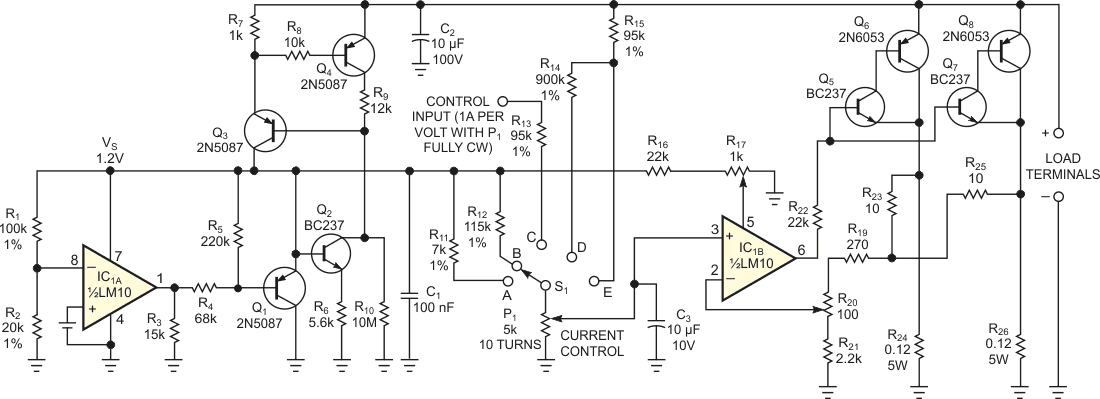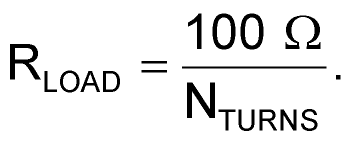# Precision active load operates as low as 2V

## Texas Instruments LM10

EDN

This Design Idea presents a self-powered, precision-active-load circuit that improves on a previously published design (Reference 1). Added features include a wider operating-voltage range of 2 to 50 V or higher and several flexible current-setting modes. The circuit in Figure 1 uses Texas Instruments' LM10, which suits this application. The LM10's reference section, IC1A, generates a precision 1.2 V reference voltage, VS. Resistive divider R1 and R2 applies a fraction of VS to IC1A's reference amplifier, which drives shunt regulator Q1.Figure 1. This versatile precision load circuit draws constant current or emulates an adjustable power resistor.

Transistor Q3 acts as a current mirror of transistor Q2's collector current and supplies power to shunt regulator Q1. Resistors R9 and R7 set the current-mirror ratio, and the current through resistor R9 depends on the current through R6, which VS establishes. As a result, Q3, which mirrors the collector current of Q2, provides power to the shunt regulator. VS sets R6, which determines the current through R9. Thus, the LM10's reference section regulates both its own power-supply voltage and the current that Q3 provides.

At power-on, Q2, Q3, and Q4 are all off. Resistor R10 draws a small amount of start-up current, which Q3 amplifies to start the current-mirror process. When sufficient current flows through R7, Q4 saturates, and R9 and R7 then set the current-mirror ratio. The active load's power-handling section comprises the LM10's operational-amplifier section, IC1B, and power transistors Q6 and Q8. A 10-turn precision potentiometer, P1, and range-selection switch, S1, set the load current as follows:

On Range A, the load current varies at 1 A per turn of P1 – that is, 10 A maximum with P1 set fully clockwise. On Range B, the load current varies at 100 mA per turn of P1 – that is, 1 A maximum with P1 set fully clockwise. On Range C, an external voltage source that connects to R13 controls the load current at a rate of 1 A per volt with P1 set fully clockwise. You can drive the external input with a function generator to test a power supply's transient response. On Range D, the load circuit emulates an adjustable power resistor with load current proportional to the voltage across the load's terminals. The equivalent resistance varies with P1's setting – that is,Range E is similar to D, with a resistance of 10 Ω/NTURNS.

To calibrate the circuit, connect it to a suitable power supply delivering any voltage from 2 to 50 V. First, set P1 to one turn – that is, one-tenth of full- scale – and S1 to Range B. Adjust R17 for a 100-mA output current. Then, rotate P1 fully clockwise and adjust R20 to set the output current to 1 A. Repeat these two adjustments in sequence because they interact slightly. Current that IC1 draws through Q3 sets the minimum current through the load circuit at slightly less than 1 mA.

Because the circuit operates at 2 to 50 V, it is suitable for testing the low-voltage outputs of a PC's power supply. You can extend the maximum voltage by selecting suitable transistors for Q2, Q3, and Q5 through Q8; the LM10's regulated power-supply voltage does not link to the external voltage. Note that when dissipating large amounts of power, transistors Q6 and Q8 require adequate cooling to maintain safe junction temperatures.

## Reference

1. Toffoli, Tommaso, "Self-powered dummy load checks out multiple power supplies," Electronic Design, April 17, 2000, pg 118.

EDN